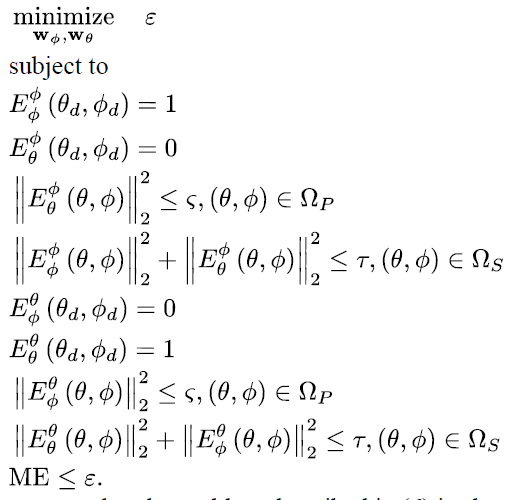# How to describe the constraints for all values ​​in the domain?Hello. I need to solve this problem. I do not know how to describe (in cvx) constraints represented in 5, 6, 9, 10 lines. Specifically I have function E, which depends on complex variables W and angular values theta and phi, for all theta and phi, such that a < phi < b and c < theta < d, E<sigma must be fulfilled.

There is one more question. How to solve this problem, if the function E will include trigonometric (sin, cos) functions? When I tried to work with them earlier in CVX, I got an error.

You haven’t provided enough information…What is E? if that is a nonlinear function of the optimization (CVX) variables, then the problem is non-convex.

As you have literally written the problem (perhaps you have typos), the problem is infeasible, because you are (twice) constraining the same thing to be both 1 and 0.

sin and cos are non-convex. Therefore they can not be applied to CVX (optimization) variables. A two term Taylor expansion of cos could be used as a concave function, but may not necessarily suit your purposes.

Here is part of the article that describes the purpose of the function E.

If necessary, I can send you the entire contents of the article. It is called “Synthesis of Conformal Arrays With Matched Dual-Polarized Patterns”.
Specifically in my case, it is necessary to apply this method to a cylindrical antenna array.
And as far as I know the function E_theta_mtheta / mphi is equal to E = Utheta*cos(phi)sin(theta), where UTheta - unit vector, similary E_phi_mtheta / mphi = Uphicos(phi)sin(theta)+Uthetasin(phi)*cos(theta).

If E involves trig functions of optimization variables, then an equality constraint involving it is non-convex, and can not be handled by CVX.

As for my above statement, “As you have literally written the problem (perhaps you have typos), the problem is infeasible, because you are (twice) constraining the same thing to be both 1 and 0”, I see now that the four equality constraints are with the four different combinations of phi and theta as subscript and superscript, so they are apparently not infeasible. Nevertheless, this appears to be non-convex.

If there is a convex optimization problem, then it’s you’ responsibility to extract it out of the paper and present it in a self-contained way. And of course you should show it is convex. If you can do all that, then maybe we can help you figure out if there is a way of entering it into CVX. Please read Why isn’t CVX accepting my model? READ THIS FIRST! .